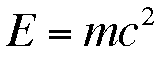# Article wrapper

List of Figures

## Chapter 1. Equations

blah blah blah.Equation 1.1First Equation

blah blah blah.blah blah blah.Equation 1.2Second EquationFigure 1.1A Figure